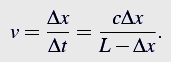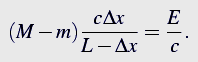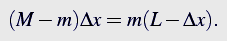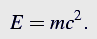A shortcut to E=mc2 14 March 2008

Have you ever considered how to actually derive the famous E=mc2  formula? Does it require knowledge of Special Relativity or even worse: an understanding of the theory?.

Well, no!
The only skill needed is some high-school physics and a bit of curiosity.

There is a box of mass M and horizontal length L in the picture below.The center of mass of the box in rest hangs somewhere above point ×c.To derive the formula it is enough to answer the question: What will happen to the center of mass position if a photon is emitted from the left wall of the box, picture a), and caught by the right wall, as in the picture b)?

Lets assume that the photon has energy E. It is known¹, that photons propagate with the speed of light c and carry momentumFrom the momentum conservation principle, after the photon emission, the box should move leftwards with some velocity v. After the photon is absorbed the box must stop due to the momentum conservation.

The velocity v is calculated in the following manner.The box moves the distance Δx to the left before the photon hits the right wall. Time of flight of the photon Δt is given byThus, the mean velocity v of the boxSo far, so good. But what has happened to the box’s center of mass? Initially it has been positioned over the point ×c, then the box moved leftwards and stopped. Did the center of mass move as well? Of course, not. There was no external force acting on the system, so the center of mass remained at the initial position.

Summarizing, there are two undeniable facts
1. The box moved,
2. Its center of mass did not.

The only way out of the apparent contradiction is to admit, that besides the energy and momentum the photon carried some mass m from the left to the right wall. (Notice, thatm is not the rest mass of the photon. Photons have zero rest mass.)

From the momentum conservation, the magnitudes of momenta of the box and photon are equalSubstituting the previously evaluated velocity v in the expression above one getsFor the center of mass to remain still, the mass displacement of the box must be balanced by displacement of the mass carried by the photon.From the two last equations one obtainsOr in more recognizable form:Sanity check: Has any Special Relativity been used in this derivation?

The original idea, similar to the box example, comes from A. Einstein (1906).

¹ The dispersion relation for light is derived from Maxwell’s equations in vacuum. First convert them to a wave equation for the magnetic field B and then try a solution B=B0cos(kx-wt).

Zbigniew Karkuszewski, March 14-th 2008